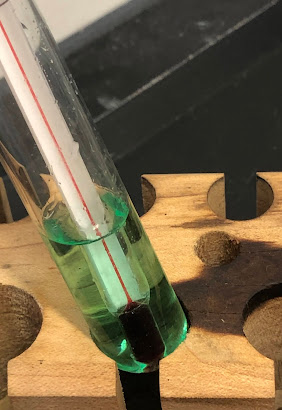## Tuesday, September 1, 2020

### Temperature Scales

Where are we going with this?
The point of this deck is to provide concepts,background information and examples related to the kinetic theory of matter. Using this model will help predict behaviors of substances within a variety of environments and help quantify

Temperature Scales
Temperature is measured using a thermometer. "Thermo" is a greek word for… just say heat. "Meter" is from a Greek word meaning measure. Hence… Never mind.Temperature is the average kinetic energy of the molecules (particles) of a substance. So why don't we just say things like, "Wow! It sure is hot! I bet the average kinetic energy of the air molecules is… like 20 N m2/s2!"

Because that would be weird. Also, I just made up the 20.

But, it's nice to remember that kinetic energy is found with the equation:

Ek = 1/2 mv2

Instead, we say that the temperature is some degree of hot. Higher degrees mean higher whatever, so in temperatures, higher degrees mean higher kinetic energy.

The degree of hotness (kinetic energy) is described using three common scales: Fahrenheit, Celsius, and Kelvin.

Fahrenheit isn't used much in science, but arguably, it could be.

Celsius was devised such that water freezes at 0° C and boils at 100° C.

Kelvin was devised based on… So… hang on for this…

If temperature is based on the average kinetic energy of the molecules as they move around in the substance, and if kinetic energy is based on how fast something is moving, then what happens if the molecules slow down? That means the temperature goes down, because… math.

What happens if the molecules slow down a lot? That means the temperature goes down a lot.

What happens if the molecules stop! That means that (look above for the equation) the energy is zero.

So, that means there is an absolute low to which energy can go, which also means there is an absolute low for temperature. The Kelvin scale sets that point at zero. Then, it uses degrees that are the same size as the Celsius degrees.

And what is this zero point on the Kelvin scale? About -273° C.

Okay, good talk.

You can use math or Google to convert from any of the scales.

If you do, here's a table.

 Celsius Kelvin Fahrenheit Boiling Water 100 373 212 Freezing Water 0 273 32 Absolute Zero -273 0 -459.67

Note: Absolute Zero is actually 273.15° K below 0° C.